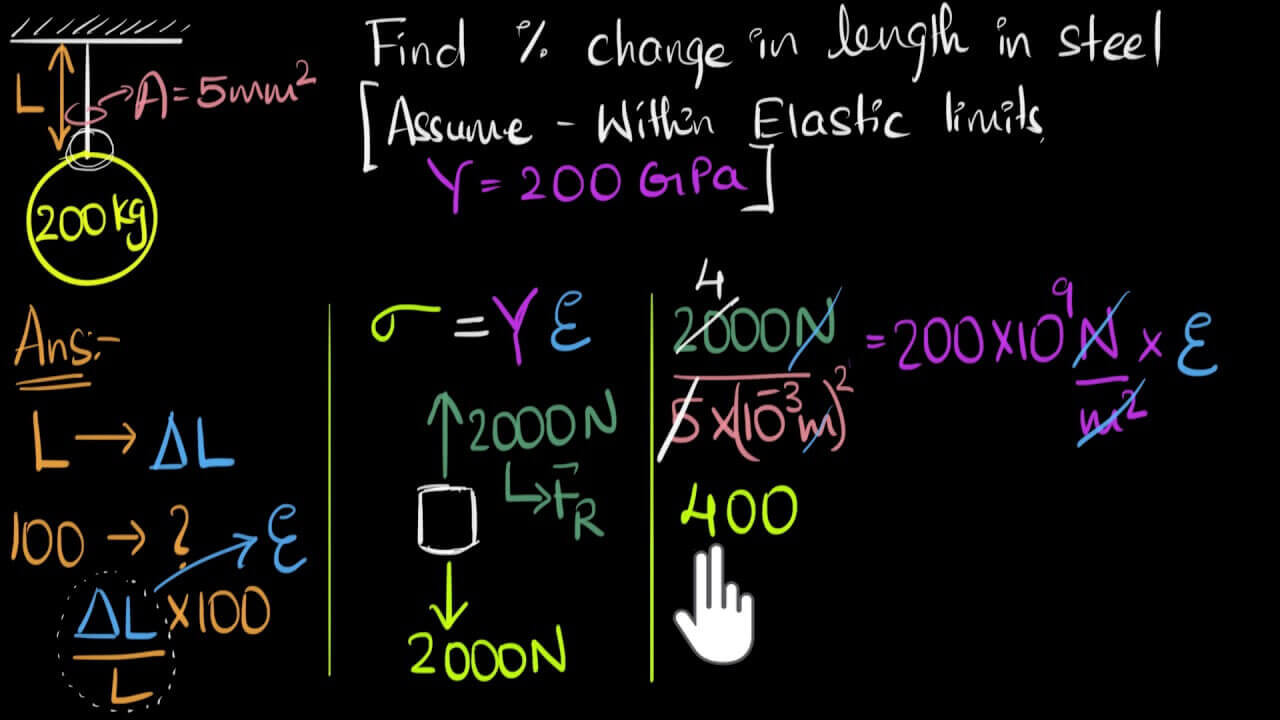# The Principle of Constant Tension Control of Slitting MachineThe essence of constant tension control in the winding and unwinding process is to know the change of roll diameter in the operation of the load, because of the change of roll diameter, in order to maintain the operation of the load, the splitter needs the output torque of the motor to follow the change of roll diameter.

The V series inverter provides three analog input ports, AUI, AVI and ACI, which can be defined as multiple functions, so that any one of them can be selected as the torque setting and the other as the speed limit. 0~10v corresponds to the inverter output of 0~motor The 0~10v corresponds to the inverter output of 0~motor rated torque, so that the control of constant tension can be completed by adjusting the 0~10v voltage.

As for the Duyu slitting machine, the part of calculating the roll diameter is calculated by the tension controller, but of course there is no problem to use PLC architecture to realize it. That is to say, you can set the tension on the man-machine or text, and calculate the roll diameter through PLC, T=F*D/2, so you can calculate the size of the torque that needs to be output from the motor, and then through the analog output to the torque giving end of the V series inverter can be.### Calculation of tension and torque

If F*D/2=T/i (F-tension, D-roll diameter, T-torque, i-reduction ratio), = F=2*T*i/D for 3.7KW AC motor, its rated torque is calculated as follows: T=9550*P/n=T= 24.88N.m so Fmax=2*24.88*9/0.6=74.64N (T-rated torque of the motor, P-rated power of the motor, n==rated speed of the motor).

## Recent Posts

### Slitting Machines and Related Tension Issuescoilslitter.com
Hi,i am windy,we are a professional manufacturer to produce slitting line and cut to length line ,what can i do for you?